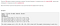# String Matching Algorithms

Write summaries of KMP Algorithm, Boyer-Moore string-search algorithm, Rabin-Karp Algorithm

# Problem Statement

Given a search string `N='ABCDEFGHIJKLMNOPQRSTUVWXYZ'` and a pattern `M='DEF'` , we want to see if the pattern `M` can be found in the string `N`.

# Naive Approach

The Naive implementation of the search algorithm will compare the pattern `M` against all possible substrings of that length in the search string `N`.

Therefore, the worst case time complexity is `O(M*N)` where the complete search space represents performing a comparison search in `O(M)` across all substrings in `O(N)` time.

This gives a time complexity which is equal to the product of the two strings.

# Background

The Largest Divisible Subset problem is a great problem which embodies many critical concepts to more advanced interviewing problems such as Dynamic Programming and Backtracking. The high-level reason why Dynamic Programming is useful in the solution is that we are asked to find the maximum of a given computation. Many times, when a problem asks to optimize, this is a perfect candidate for Dynamic Programming. The high-level reason why Backtracking is useful in this problem is that we will be optimizing the subset length, while the problem asks us to return the resulting subset whose length is this optimal value…

# Background

The Knapsack problem, according to Wikipedia, is one of the most studied problems in combinatorial optimization.

The general description of the knapsack problem is the following:

• Given a set of `n` items, where each item has an associated profit `p_j` and a corresponding weight `w_j` , perform a series of binary decisions to select a subset of items such that the profit is maximized while the cost is kept within constraints.

The name `Knapsack` stems from the problem faced by someone who is picking items to put in his knapsack such that he fills the bag with the “best” items…

# Background

I have been intrigued by Leet Code style questions for a while. I have been very inspired by channels like Back to Back SWE, who goes beyond the code to develop a deep understanding of what is happening.

This is my attempt to give back.

# Problem StatementTwo City Scheduling — Prompt

The Two City Scheduling problem requires that you fly out `2N`people equally into 2 different cities, such that both cities have `N` people. Differing costs are incurred by placing a person in either city. Thus, you want to design an algorithm that seeks the minimum cost.

# Approach

While Leet Code defines this problem as Easy, it…